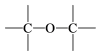Search:

# General organic formulas

1 Give the formulas of molecules with only the following features: a) a ring and a double bond b) two cycles, a carboxylic acid functional group c) a triple bond, a keto functional group and an amine functional group

2 Give the molecular formula of molecules with only the following features: a) 5 C atoms, two cycles b) 8 C atoms, three double bonds, an ester function

3 A monocyclic dialcohol has one multiple bond as a double bond. Its molecular weight is 114. Find its molecular formula by an algebraic calculation.

4 A non-cyclic substance has the molecular formula C3H6 O. Find all possible features (functions, multiple bonds, cycles .. ) of this molecule, for example: a) a double bond and an alcohol or b) ....... or c) ....... Note: the functional groupis called ether